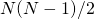March 13, 2017

# Meta-learning Algorithms

Learning approaches that rely on another algorithm performing some enrichment, variation, or generalization.

### OneVsAllLearning

Java classOneVsAllLearning

Source codeOneVsAllLearning.java

Maven Project: kelp-core

JSON type: oneVsAll

Description: it is a meta algorithm that operates applying a One-Vs-All strategy over the base learning algorithm which is intended to be a binary learner. The One-Vs-All strategy will learn N different classifiers, where N is the number of classes involved in the dataset. In this strategy each classifier is learned by considering in turn the examples of a single class as positives, while all the other examples are considered as negative.

Parameters:

• baseAlgorithm: The base learning algorithm which is intended to be a binary learner
• labels: The list of targeted classes

### OneVsOneLearning

Java classOneVsOneLearning

Source codeOneVsOneLearning.java

Maven Project: kelp-core

JSON type: oneVsOne

Description: it is a meta algorithm that operates by applying a One-Vs-One strategy over the base learning algorithm which is intended to be a binary learner. This meta-algorithms will learnclassifiers, by comparing each class with all the others separately. The resulting classifier applies a voting strategy to perform the final decision. (N is the number of classes in the dataset)

Parameters:

• baseAlgorithm: the base learning algorithm which is intended to be a binary learner
• labels: the list of targeted classes

### MultiLabelClassificationLearning

Java classMultiLabelClassificationLearning

Source codeMultiLabelClassificationLearning.java

Maven Project: kelp-core

JSON type: multiLabel

Description: it is a meta algorithm that operates applying a multi label learning strategy over the base learning algorithm which is intended to be a binary learner. A multi label classification task is a multiclass problem in which each instance can belongs to zero, one or multiple classes. The multi label strategy will learn N different classifiers, where N is the number of classes involved in the dataset. In this strategy each classifier is learned by considering in turn the examples of a single class as positives, while all the other examples are considered as negative.

Parameters:

• labels: the list of targeted classes
• baseAlgorithm: the base learning algorithm which is intended to be a binary learner

### MultiEpochLearning

Java classMultiEpochLearning

Source codeMultiEpochLearning.java

JSON type: multiEpoch

Description: it is a meta learning algorithms for online learning methods. It performs multiple iterations (or epochs) on the training data.

Parameters:

• baseAlgorithm: The base online learning algorithm which is intended to apply for multiple iterations
• epochs: The number of iterations

### Stoptron

Java class: Stoptron

Source codeStoptron.java

JSON type: stoptron

Description: it is a variation of the Stoptron proposed in [Orabona et al.(2008)]. Until the budget is not reached the online learning updating policy is the one of the baseAlgorithm that this meta-algorithm is exploiting. When the budget is full, the learning process ends.

Parameters:

• label: The label to be learned
• baseAlgorithm: The base online learning algorithm which is intended to apply in this budgeted approach
• budget: The maximum number of support vectors allowed in the budget

### RandomizedBudgetPerceptron

Java classRandomizedBudgetPerceptron

Source codeRandomizedBudgetPerceptron.java